#### You may also like### Pizza Portions

My friends and I love pizza. Can you help us share these pizzas equally?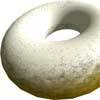### Doughnut

How can you cut a doughnut into 8 equal pieces with only three cuts of a knife?### Pies

Grandma found her pie balanced on the scale with two weights and a quarter of a pie. So how heavy was each pie?

##### Age 7 to 11Challenge Level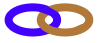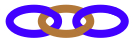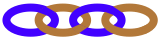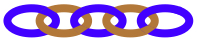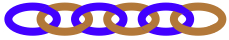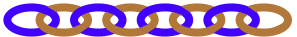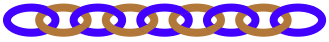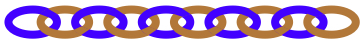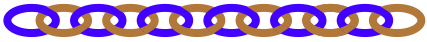Here are lots of small chains, each with a certain number of links.  The chains that we will use have these numbers of links:  2, 3, 4, 5, 6, 8, 9, 10 and 12. There are many of each size available.

Suppose we want to make a twenty-link chain from the sizes above, then we could use two of the ten-link small chains. Each ten would just be $\frac{1}{2}$ of the 20.
In this case we could write:

Half plus half makes one whole, or, $\frac{1}{2} + \frac{1}{2} = 1$
where the twenty-link chain is the ONE (1)

What other selection of chains could you put together to make the twenty-link chain, using only the sizes that you see at the top?
Try writing the fractions down in different ways.

Find as many different ways of making a twenty-link chain as you can.

What happens if you try to make a twenty-four-link chain?

Here is an example using a twelve-, an eight- and a four-link chain:

We could write:
$\frac{1}{2} + \frac{1}{3} + \frac{1}{6} = 1$
where the twenty-four-link chain is the ONE (1)

Or, we could write:
a half plus a third plus a sixth makes a one

How many ways can you find to make a twenty-four-link chain?

A very different one to try is a twenty-seven-link chain, so 27 is your ONE.  Have a go!